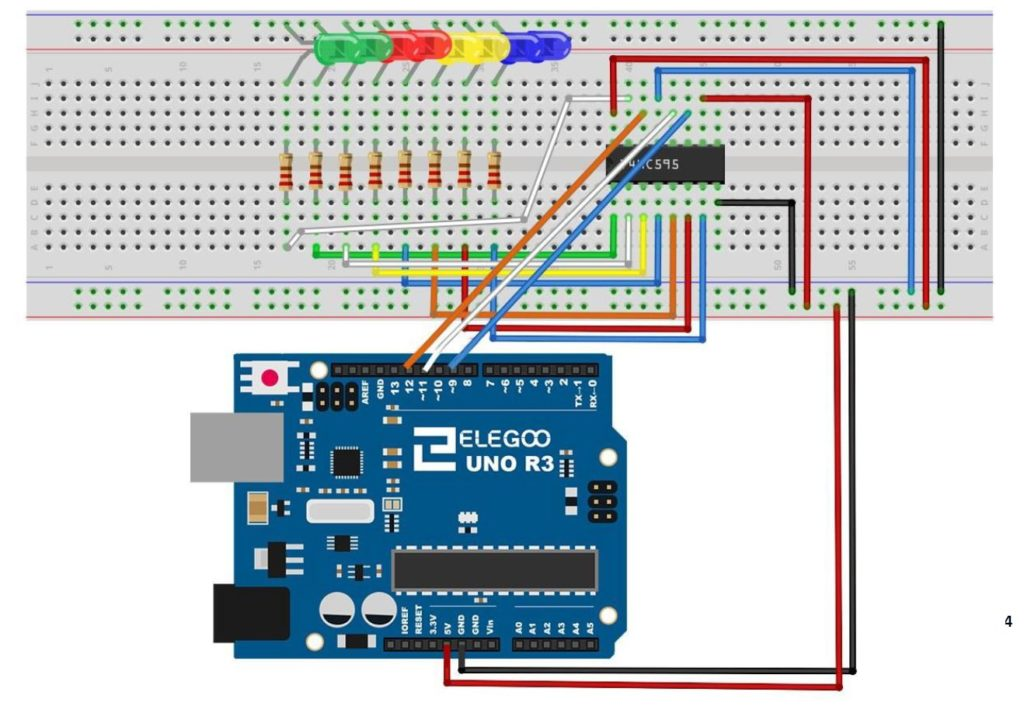# Arduino Tutorial 47: Binary and Hexadecimal Bit Flipper

In this lesson we explore how to create a Binary or Hexadecimal Bit Flipper. From our earlier lessons you see we can visually represent Hexadecimal or Binary numbers with a series of LED, with an on LED representing a “1” and an off LED representing a “0”. In programming and circuit applications, it is sometimes useful to “flip” or invert the bits. For an 8 bit number, one could do this in a program with 255 IF statements, but there is a simpler way. If you think about it, you can get the flippedByte by simple subtracting the byte from 0xFF, or 0b11111111. If you try some test cases, you can see that this will always work.

Simply stated, flippedByte=0xFF-Byte,

or if you prefer thinking in binary,

flippedByte=0b11111111-Byte

This is the circuit we are using to drive the 8 LED with the 74HX595 chip, and all this was explained in Tutorial 42.This is the schematic we use in this example to control 8 LEDs from the 74HC595 chip.

This is the code which we developed in the video above.

In all these lessons we are using the Arduino Super Starter Kit, which you can pick up HERE.

# Arduino Tutorial 40: Controlling DC Motor Speed and Direction with Pushbuttons

In this lesson we explore how to control the speed and direction of a DC motor using two buttons. We are using the L293D motor controller and a small DC motor for demonstration purposes. We are using parts from our Elegoo Super Stater Kit, which you can get HERE. The basic circuit was explained in Lesson 37, and we are using that work as a starting point. The schematic below will get you started in connecting your circuit. Be sure and connect one of the Arduino ground pins to the ground rail in the second to the bottom row in the diagram below. It is good practice to connect all your grounds together.

The code we developed in the video lesson is shown below for your convenience.

# Arduino Tutorial 35: Understanding How to Use a Stepper Motor

In this lesson we give you several examples of how to connect and program a stepper motor. Stepper motors are useful because they can produce very large torque at low RPM and are capable of extremely precise positioning. They are somewhat tricky to use, and you must be careful to not try and power them from an Arduino. Arduino can control stepper motors, but they must be powered from an external power supply.

If you want to follow along at home, you can order the Arduino Kit we are using HERE.

This is the code that allows you to toggle the direction of the stepper motor by pressing a pushbutton. The video shows all the details and how to connect the motor up.

# Arduino Tutorial 24: Understanding Passive Buzzers

In this lesson we show you how to incorporate sound into your project using a passive buzzer. Passive Buzzers have the advantage that they are less expensive than active buzzers, and you can more precisely control the tone. This lesson will show you how to hook up the buzzer, and then how to code the arduino to produce different tones.

If you want to follow along at home, you can order the Arduino Kit we are using HERE.